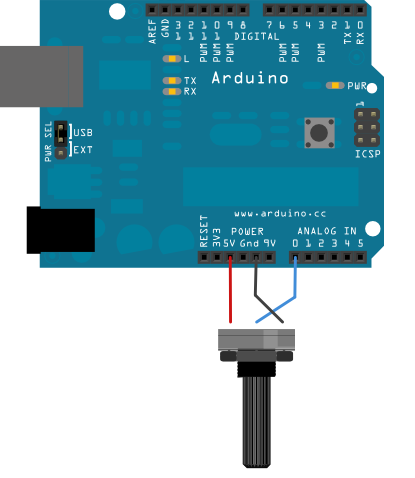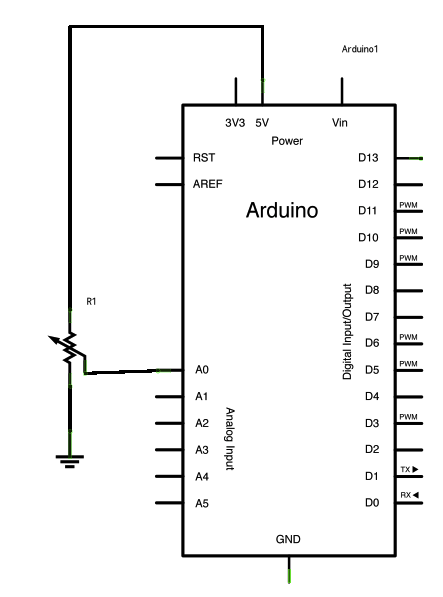## If Statement (Conditional Statement)

The if() statement is the most basic of all programming control structures. It allows you to make something happen or not, depending on whether a given condition is true or not. It looks like this:

```if (someCondition) {
// do stuff if the condition is true
}

```

There is a common variation called if-else that looks like this:

```if (someCondition) {
// do stuff if the condition is true
} else {
// do stuff if the condition is false
}

```

There's also the else-if, where you can check a second condition if the first is false:

```if (someCondition) {
// do stuff if the condition is true
} else if (anotherCondition) {
// do stuff only if the first condition is false
// and the second condition is true
}

```

You'll use if statements all the time. The example below turns on an LED on pin 13 (the built-in LED on many Arduino boards) if the value read on an analog input goes above a certain threshold.

### Hardware Required

• Arduino or Genuino Board
• Potentiometer or variable resistor

### Circuit

click the image to enlargeimage developed using Fritzing. For more circuit examples, see the Fritzing project page

### Schematic

click the image to enlarge### Code

In the code below, a variable called `analogValue` is used to store the data collected from a potentiometer connected to the board on analogPin 0. This data is then compared to a threshold value. If the analog value is found to be above the set threshold the built-in LED connected to digital pin 13 is turned on. If analogValue is found to be `<` (less than) threshold, the LED remains off.